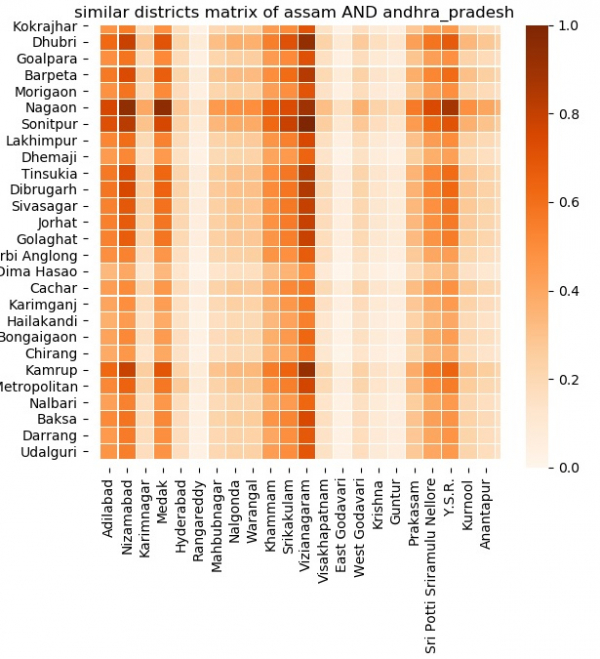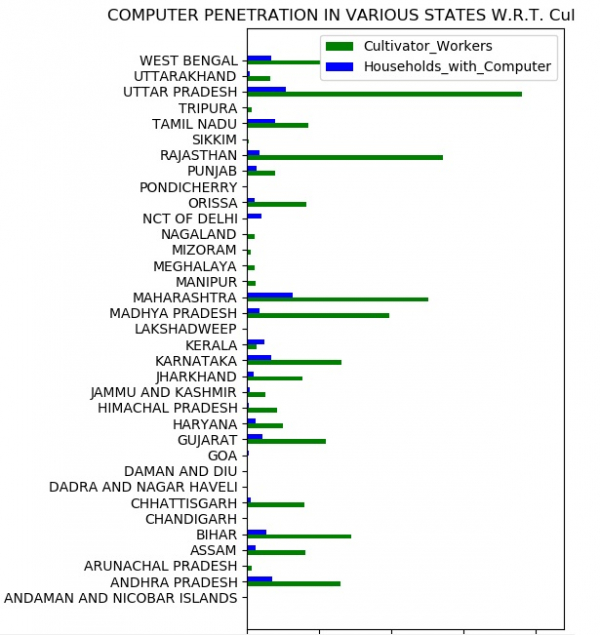# Analyzing Census Data in Python

PythonServer Side ProgrammingProgramming

Census is about recording information about a given population in a systematic manner. The data captured includes various category of information like – demographic, economic, habitation details etc. This ultimately helps the government in understanding the current scenario as well as Planning for the future. In this article we will see how to leverage python to analyze the census data for Indian population. We will look at various demographic and economic aspects. Then plot charge which will project the analysis in a graphical manner. The source that is collected from kaggle. It is located  here.

## Organizing the Data

In the below program first we acquire the data using a short python program. It just loads the data to the pandas dataframe for further analysis. The output shows some of the fields for simpler representation.

## Example

import pandas as pd
#https://www.kaggle.com/danofer/india-census#india-districts-census-2011.csv
print(datainput)

## Output

Running the above code gives us the following result −

      District code ... Total_Power_Parity
0                 1 ...               1119
1                 2 ...               1066
2                 3 ...                242
3                 4 ...                214
4                 5 ...                629
..              ... ...                ...
635             636 ...              10027
636             637 ...               4890
637             638 ...               3151
638             639 ...               3151
639             640 ...               5782

[640 rows x 118 columns]

## Analysing Similarity Between Two States

Now that we have gathered the data we can proceed to analyze the similarities on various fronts between two States. The similarities can be on the basis of age group, computer ownership, housing availability, education level etc. In the below example we take the two states named Assam and Andhra Pradesh. Then we compare the two states using the similarity_matrix. All the data fields are compared for each possible pair of districts from both the states. The resulting heatmap indicates how closely these two are related. The darker the shade the closer they are related.

## Example

import pandas as pd
import matplotlib.pyplot as plot
from matplotlib.colors import Normalize
import seaborn as sns
import math
df_ASSAM = datainput.loc[datainput['State name'] == 'ASSAM']
def segment(x1, x2):
# Set indices for both the data frames
x1.set_index('District code')
x2.set_index('District code')
# The similarity matrix of size len(x1) X len(x2)
similarity_matrix = []
# Iterate through rows of df1
for r1 in x1.iterrows():
# Create list to hold similarity score of row1 with other rows of x2
y = []
# Iterate through rows of x2
for r2 in x2.iterrows():
# Calculate sum of squared differences
n = 0
for c in list(datainput)[3:]:
maximum_c = max(datainput[c])
minimum_c = min(datainput[c])
n += pow((r1[c] - r2[c]) / (maximum_c - minimum_c), 2)
# Take sqrt and inverse the result
y.append(1 / math.sqrt(n))
# Append similarity scores
similarity_matrix.append(y)
p = 0
q = 0
r = 0
for m in range(len(similarity_matrix)):
for n in range(len(similarity_matrix[m])):
if (similarity_matrix[m][n] > p):
p = similarity_matrix[m][n]
q = m
r = n
print("%s from ASSAM and %s from ANDHRA PRADESH are most similar" % (x1['District name'].iloc[q],x2['District name'].iloc[r]))
return similarity_matrix
normalization=Normalize()
s = plot.axes()
plot.rcParams['figure.figsize'] = (20,20)
plot.show()

## Output

Running the above code gives us the following result −## Comparing specific parameters

Now we can also compare places with respect to specific parameters. In the below example we compare the availability of household computers available for the cultivator workers. We produce graph which shows the comparison between these two parameters for each of the state.

## Example

import pandas as pd
import matplotlib.pyplot as plot
from numpy import *

z = datainput.groupby(by="State name")
m = []
w = []
for k, g in z:
t = 0
t1 = 0
for r in g.iterrows():
t += r
t1 += r
m.append((k, t))
w.append((k, t1))
mp= pd.DataFrame({
'state': [x for x in m],
'Households_with_Computer': [x for x in m],
'Cultivator_Workers': [x for x in w]})

d = arange(35)
wi = 0.3
fig, f = plot.subplots()
plot.xlim(0, 22000000)
r1 = f.barh(d, mp['Cultivator_Workers'], wi, color='g', align='center')
r2 = f.barh(d + wi, mp['Households_with_Computer'], wi, color='b', align='center')
f.set_xlabel('Population')
f.set_title('COMPUTER PENETRATION IN VARIOUS STATES W.R.T. Cultivator_Workers')
f.set_yticks(d + wi / 2)
f.set_yticklabels((x for x in mp['state']))
f.legend((r1, r2), ('Cultivator_Workers', 'Households_with_Computer'))
plot.rcParams.update({'font.size': 15})
plot.rcParams['figure.figsize'] = (15, 15)
plot.show()

## Output

Running the above code gives us the following result −